• 2020-11-11 16:42:14

### 线性方程组、方程组解的结构

手动反爬虫： 原博地址

 知识梳理不易，请尊重劳动成果，文章仅发布在CSDN网站上，在其他网站看到该博文均属于未经作者授权的恶意爬取信息


如若转载，请标明出处，谢谢！

# 1 线性方程组

最熟悉的鸡兔同笼的问题，假使鸡兔共八只，腿共20条，请问有多少鸡和兔子？

古人思路：
1）抬脚法：兔子抬起两只脚，那么鸡兔共16条腿，剩下4腿就是兔子的，所以兔子2只，鸡6只
2）落脚法：假使鸡有四条腿，都落下来，鸡兔共32条腿，多的12条腿就是鸡的，所以鸡6只，兔子2只

如果使用现代的方程组的思路就是：设鸡有 x x 只，兔子 y y 只，方程组为： { x + y = 8 2 x + 4 y = 20 ⇒ { x = 6 y = 2 \begin{cases} x+y =8 \\ 2x + 4y=20 \end{cases} \Rightarrow \begin{cases} x=6 \\ y=2 \end{cases}

方程的运算（消元法）基本步骤：
1）交换两方程
2）用非零数乘其方程
3）某方程的 l l 倍加到另一方程
可以发现消元法解方程就对应着三种初等行变换，因此上面的方程组形式就可以简化一下使用矩阵进行表示，比如计算过程如下
( 1 1 8 2 4 20 ) ⇒ ( 1 1 8 0 1 2 ) ⇒ ( 1 0 6 0 1 2 ) \left(\begin{matrix} 1&1&8\\2&4&20\end{matrix}\right)\Rightarrow \left(\begin{matrix} 1&1&8\\0&1&2\end{matrix}\right)\Rightarrow \left(\begin{matrix} 1&0&6\\0&1&2\end{matrix}\right)

# 2 方程组有解的判定

## 2.1 方程组的向量和矩阵表示

假使方程组如下，可以转化为向量的表示形式
{ x 1 + x 2 + x 3 = 1 x 1 − x 2 − x 3 = − 3 2 x 1 + 9 x 2 + 10 x 3 = 11 ⇒ x 1 ( 1 1 2 ) + x 2 ( 1 − 1 9 ) + x 3 ( 1 − 1 10 ) = ( 1 − 3 11 ) ⇒ x 1 α 1 + x 2 α 2 + x 3 α 3 = β \begin{cases} x_{1}+x_{2}+x_{3} =1 \\ x_{1}-x_{2}-x_{3} =-3\\ 2x_{1}+9x_{2}+10x_{3} = 11 \end{cases} \Rightarrow x_{1}\left(\begin{matrix} 1\\1\\2\end{matrix}\right)+x_{2}\left(\begin{matrix} 1\\-1\\9\end{matrix}\right)+x_{3}\left(\begin{matrix} 1\\-1\\10\end{matrix}\right) = \left(\begin{matrix} 1\\-3\\11\end{matrix}\right)\Rightarrow x_{1}\alpha_{1}+x_{2}\alpha_{2}+x_{3}\alpha_{3}=\beta 矩阵表示形式：系数矩阵就为方程组变量的所有系数构成的矩阵，记作 A A ，增广矩阵就是在系数矩阵的基础上添加常数项，记作 A ‾ \overline{\text{A}}
A = ( 1 1 1 1 − 1 − 1 2 9 10 )    A ‾ = ( 1 1 1 1 1 − 1 − 1 − 3 2 9 10 11 ) A = \left(\begin{matrix} 1&1&1\\1&-1&-1\\2&9&10\end{matrix}\right)\space \space \overline{\text{A}}= \left(\begin{matrix} 1&1&1&1\\1&-1&-1&-3\\2&9&10&11\end{matrix}\right) 使用消元法进行方程组的求解，实际上就是对增广矩阵进行初等变换

## 2.2 方程组解的判定

假使最后化简的增广矩阵的式子如下
( 1 0 0 1 0 1 0 2 0 0 1 3 ) ⇒ { x 1 = 1 x 2 = 2 x 3 = 3 ⇒ 方 程 组 有 唯 一 解 ， r ( A ) = r ( A ‾ ) = 3 = 未 知 数 个 数 \left(\begin{matrix} 1&0&0&1\\0&1&0&2\\0&0&1&3\end{matrix}\right) \Rightarrow \begin{cases} x_{1}=1 \\ x_{2} =2\\ x_{3} = 3 \end{cases} \Rightarrow 方程组有唯一解，r(A)=r(\overline{\text{A}})=3=未知数个数
( 1 0 1 5 0 1 1 9 0 0 0 0 ) ⇒ { x 1 = 5 − x 3 x 2 = 9 − x 3 ⇒ 方 程 组 无 穷 多 解 ， r ( A ) = r ( A ‾ ) = 2 < 未 知 数 个 数 \left(\begin{matrix} 1&0&1&5\\0&1&1&9\\0&0&0&0\end{matrix}\right) \Rightarrow \begin{cases} x_{1}=5-x_{3} \\ x_{2} =9-x_{3}\\ \end{cases} \Rightarrow 方程组无穷多解，r(A)=r(\overline{\text{A}})=2<未知数个数
( 1 0 1 5 0 1 1 9 0 0 0 1 ) ⇒ { x 1 = 5 − x 3 x 2 = 9 − x 3 0 = 1 ⇒ 方 程 组 无 解 ， r ( A ) < r ( A ‾ ) \left(\begin{matrix} 1&0&1&5\\0&1&1&9\\0&0&0&1\end{matrix}\right) \Rightarrow \begin{cases} x_{1}=5-x_{3} \\ x_{2} =9-x_{3}\\ 0 =1 \end{cases} \Rightarrow 方程组无解，r(A)<r(\overline{\text{A}})

结论：
1）当 r ( A ) = r ( A ‾ ) r(A)=r(\overline{\text{A}}) ，方程组有解 { r ( A ) = r ( A ‾ ) = n , 唯 一 解 r ( A ) = r ( A ‾ ) < n , 无 穷 多 解 \begin{cases} r(A)=r(\overline{\text{A}})=n,唯一解\\ r(A)=r(\overline{\text{A}})<n,无穷多解\\ \end{cases}
2）当 r ( A ) ≠ r ( A ‾ ) r(A)\not=r(\overline{\text{A}}) ，方程组无解

★★★★★关于方程组中的 m , n m,n ，其中 m m 是指方程的个数， n n 是指未知数的个数

解题步骤：

1）写出增广矩阵 A ‾ \overline{\text{A}}

2）只做初等行变换，化为阶梯型矩阵

3）对比 r ( A ) , r ( A ‾ ) r(A),r(\overline{\text{A}}) 值，阶梯型中虚线左边非零行行数与右边的非零行行数的对比

4）化为行最简阶梯型，不管零行，非零行的首非零元留在左边，其余变量挪到右边得到一般解（要变号）

例题，经过转化后的增广矩阵和求解过程如下
A ‾ = ( 1 2 3 1 2 0 3 7 − 1 3 0 0 0 0 0 0 0 0 0 0 ) ⇒ ( 1 0 − 5 3 5 3 0 0 1 7 3 1 3 1 0 0 0 0 0 0 0 0 0 0 ) ⇒ { x 1 = 5 3 x 3 − 5 3 x 4 x 2 = 1 − 7 3 x 3 + 1 3 x 4 \overline{\text{A}} = \left(\begin{matrix} 1&2&3&1&2\\0&3&7&-1&3\\0&0&0&0&0\\0&0&0&0&0\end{matrix}\right) \Rightarrow \left(\begin{matrix} 1&0&-\frac{5}{3}&\frac{5}{3}&0\\0&1&\frac{7}{3}&\frac{1}{3}&1\\0&0&0&0&0\\0&0&0&0&0\end{matrix}\right)\Rightarrow \begin{cases} x_{1} = \frac{5}{3}x_{3}-\frac{5}{3}x_{4}\\ x_{2} = 1-\frac{7}{3}x_{3} +\frac{1}{3}x_{4}\\ \end{cases}matrix
更多相关内容
• 如果线性方程组有解(齐次...齐次方程组解的线性组合仍然是齐次方程组的解。 非齐次方程组： 使用消元法后，令所有的自由变量对应的未知数取0，求解主元变量对应的未知数的值，可以获得一个特解。非齐次方程组的通解...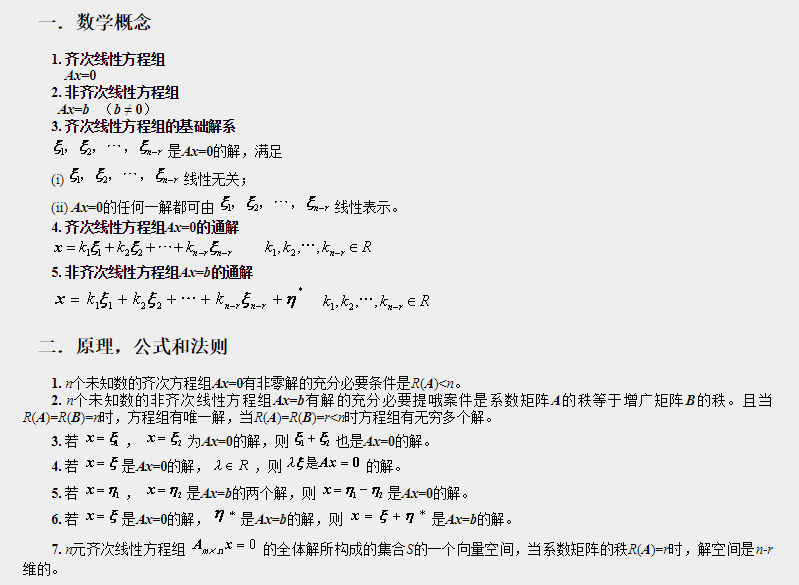如果线性方程组有解(齐次的存在非零解),则解的结构总结如下：
齐次方程组： 使用消元法后，分别对每一个自由变量对应的未知数取1，其他自由变量取对应的未知数0，可以获得齐次方程组的线性无关的特解，构成齐次方程组的基础解系。齐次方程组解的线性组合仍然是齐次方程组的解。
非齐次方程组： 使用消元法后，令所有的自由变量对应的未知数取0，求解主元变量对应的未知数的值，可以获得一个特解。非齐次方程组的通解是特解加上齐次方程组的线性组合。

# 3 解的判定

齐次线性方程组：
a11 x1 + a12 x2 + … + a1n x n = 0 ,
a21 x1 + a22 x2 + … + a2n x n = 0 ,

as1 x1 + as2 x2 + … + asn x n = 0
首先需要说明的是齐次线性方程组的解只有两种情况，只有零解和有非零解。 不存在没有解的情况。
有非零解的充分必要条件是: 它的系数矩阵的秩 r 小于未知量个数 n . 矩阵中的最大的不相关的向量的个数，就叫
上面的有非零解的条件有很多等价的条件：

1. 系数矩阵A是非奇异矩阵。有关奇异矩阵的内容参考博客奇异矩阵与非奇异矩阵
2. 系数矩阵存在线性相关的列。
3. 使用消元法之后主元的数目小于未知数的数目。

非齐次线性方程组：
a11 x1 + a12 x2 + … + a1n x n = 0 ,
a21 x1 + a22 x2 + … + a2n x n = 0 ,

as1 x1 + as2 x2 + … + asn x n = 0
非齐次线性方程组解的情况有三种：无解，唯一解和无穷多解。
有解的充分必要条件是 : 它的系数矩阵与增广矩阵有相同的秩 . 这有解包含了有无穷多解和唯一解。
如果系数矩阵与增广矩阵的值相同且等于未知量的个数n则存在唯一解。
如果系数矩阵与增广矩阵的值相同且小于未知量的个数n则存在无穷多解。
说明几点可以方便我们理解上面解的情况：
非齐次线性方程组的形式为：
A x = b Ax=b
上面式子的意思是求系数x，使得A的各列按照系数线性组合获得b。
系数矩阵与增广矩阵有相同的秩说明b与A的各列线性相关，b可以由A的各列线性表示，所以存在存在解。
系数矩阵与增广矩阵的秩不同说明b与A的各列线性无关，b不可以由A的各列线性表示，所以不存在存在解。
如果系数矩阵与增广矩阵的值相同且等于未知量的个数n 说明A是满秩的（列满秩），A的所有的列线性无关，就是不存在自由变量，b可以由A的各列按照唯一的系数表示，所有存在唯一解。
如果系数矩阵与增广矩阵的值相同且小于未知量的个数n说明A不是满秩的，就是A的有些列可以用其他列线性表示，就是存在自由变量，自由变量的取值是任意的，所以存在无穷多解。

参考博客：

展开全文• 给出了常系数线性微分方程组...以广义特征向量链、指数矩阵和矩阵的秩为工具,分3种情形讨论了重根情形下常系数线性微分方程组矩阵表示,建立了统一的代数结构,并对后2种情形,给出了相应的实例,以说明方法的有效性。
• 线性方程组结构 如何处理一些没有或是有无数的线性方程组？ 举例 三元一次方程组 高斯-约旦消元法 ==> 在将第三行与第二行相加消元后 ==> 第三行全为0，无法找到一组xyz来满足这个线性方程组，此时这...

# 线性方程组的结构

如何处理一些没有解或是有无数解的线性方程组？

举例 三元一次方程组高斯-约旦消元法 ==>
在将第三行与第二行相加消元后 ==>第三行全为0，无法找到一组xyz来满足这个线性方程组，此时这个线性方程组是无解的！

举例2 三元一次方程组高斯-约旦消元法 ==>在将第三行与第二行相加消元后 ==>在此时，第三行的方程组全为0，这个方程是成立的，存在xyz满足相乘后为0，即xyz任意取值都能成立。

在这种情况下，高斯消元的过程已经结束了。所以反向执行约旦消元法。
从最后一个主元开始进行操作，而此时的最后一个主元不在第三行而是第二行，第三行已经没有主元了。

==> 消去第二行主元上的所有元素==> 代表的方程组而此时，这个解意味着，z任意取值，都能得到一组x,y,z,满足方程组 ==> 方程组有无数组解

高斯-约旦消元法本质上是将增广矩阵 变成了 阶梯型矩阵
定义阶梯型矩阵
==>1. 如果矩阵有全零行，那全零行一定位于矩阵的最底层2. 对于其他非全零行的每一行，其中第一个非零元素（主元）随着行数的不断上升，位置逐渐向右偏
3. 非零行的第一个元素（主元）为1
4. 主元所在列的其他元素均为0

行最简形式
reduced row echelon form RREF

举例 复杂的行最简形式
满足行最简形式的定义举例 不是行最简形式通过行最简形式来理解线性方程组解的结构

1. 有唯一解2. 无解3. 有无数解综上， 系数矩阵的非零行 < 行最简形式非零行 ==> 方程组无解
A非零行 < 未知数个数 ==> 方程组无数解
反例方程数与未知数不匹配，有唯一解

A非零行 = 未知数个数 ==> 方程组唯一解
反例方程数与未知数不匹配，有无数解。

# 直观理解线性方程组解的结构

n个未知数需要有n个方程，才可能有唯一解。
举例 二元一次方程在二维平面中表示这个方程 ==>即表示，在这条直线上的任意点都可以满足这个方程。

如果再加上一个方程 ==>在二维平面中表示这两个方程 ==>即表示，在这两条直线的交点满足该方程组，有唯一解。

将第二个方程调换 ==>在二维平面中表示这两个方程 ==>即表示，这两条直线没有交点，无解。

综上 ==> n个未知数有n个方程 才有可能有唯一解，或是无解。

将上述思路拓展到 三元一次方程中 ==>将这个方程放到三维空间中 ==>使用两个三元一次方程联立 ==>如果这两个平面相交的话，即会有一条直线相交，那这条直接上所表示的所有点，无数个点，都可以满足这两个方程组。如果两个平面平行，则没有交点，无解。

综上 ==> 如果方程组的个数小于未知数个数，那就一定没有唯一解，或是无解。

引入第三个三元一次方程 ==>在三个平面都相交的情况下，就可能在两个平面相交的直线上，产生一个三个平面都相交的点，这个点就是唯一解。

==> 在另一种情况下在这种情况下，三个平面相交的是一条直线，所以有无数解。在这三个平面平行的情况下，没有交点，无解。在这种情况下，虽然有平面相交，但是相交的位置没有公共点，相交的位置是平行的，所以无解。在这种情况下，虽然三个平面两两相交，但是得到的位置是三条平行线，所以无解。

如果再引入一个三元一次方程，即四个三元方程联立会是什么情况呢？
==>在这种情况下，虽然这四个平面两两相交，但是也没有公共点，这四个平面中间存在一个立体三角形的空间。在不断的调整这个四个平面，使这个个立体三角形不断的缩小，直到只有一个点的时候，此时这个方程组就有了唯一解。

更直观的通过二元方程来看待这个问题 ==>
三个二元方程联立在这种情况下，没有公共点。只有在新的直线与之前的两个的交点 相交的时候，才有公共点。当三根直线完全重合时 ==>此时，这三个二元方程的交点为一条直线，有无数解。

综上，如果方程个数 多于 未知数个数 ⇒ 这个方程可能唯一解、无解、无数解。

这是正常情况下 ==>在化为行最简形式后 ==>
系数矩阵的非零行 不可能大于 未知数个数

不可能存在这样一个矩阵，在只有三列的系数矩阵中，却有四个非零行，同时还是一个行最简形式。综上，在行最简式情况下 ==>而其中，无解的判断非常简单。在增广矩阵的系数矩阵化为行最简形式后，看有没有存在矛盾的行就可以判断了。所以，在行最简形式下可以简化为 ==>而在 行最简形式A非零行 = 未知数 的时候，此时是不会有无数解的 ==>因为，行最简形式的定义就说明了，如果系数矩阵的 A非零行 = 未知数 的话，那非零行的系数矩阵 一定是单位矩阵。
==> 系数矩阵的非零行 = 未知数个数 ==> 一定有唯一解

所以，在行最简形式下可以简化为 ==>这个结论的前提是 系数矩阵化为行最简形式后，不存在矛盾行 。
行最简形式A非零行 < 行最简形式Ab非零行 ==> 无解

# 更一般的线性系统求解

如果 方程个数 与 未知数个数 不匹配的话，在使用高斯-约旦消元法的时候，会产生什么问题呢，怎样来处理？

举例 五元方程，四个方程组 ==>执行前向过程 ==>发现 在第二、三、四行，除了首元素化为了0，第二个元素也都化为了0，没有一个元素可以当作主元，那应该怎么办呢？
> 继续从第三个元素 确定主元> 继续执行前向操作==> 继续确定第三行主元，但是发现第三行第四列元素为0，并且下面所有的行第四列都为0，所以 第四列找不到主元 ==> 从第五列找主元==>继续执行前向操作最终化为 ==>==> 执行后向操作，第三行主元上面的元素都化为0==> 第二行主元上面的元素都化为0
==> 行最简形式对应的方程 ==>> 变换为以主元的等式方程即x,z,u符合这个线性方程组的一组解，w，y可以任意取值。
> 无数解含有主元的 称为 主元列
没有主元的 称为 自由列

将上述一组解 化为 列向量形式 ==>从行最简形式 直接读出了 整个线性系统的解。

举例 二元方程，三个方程组 ==>执行前向操作 ==>由于 最后一行矛盾 ==> 方程组无解。

展开全文•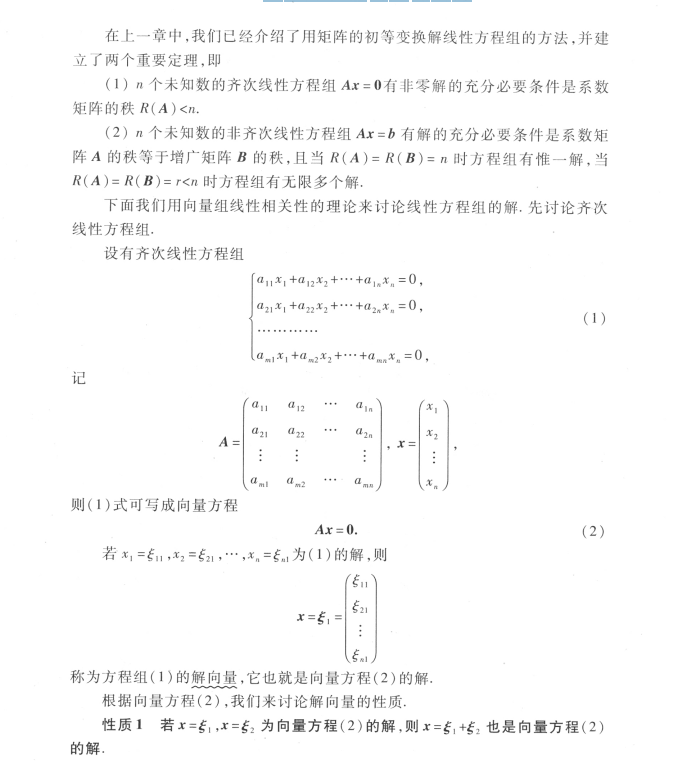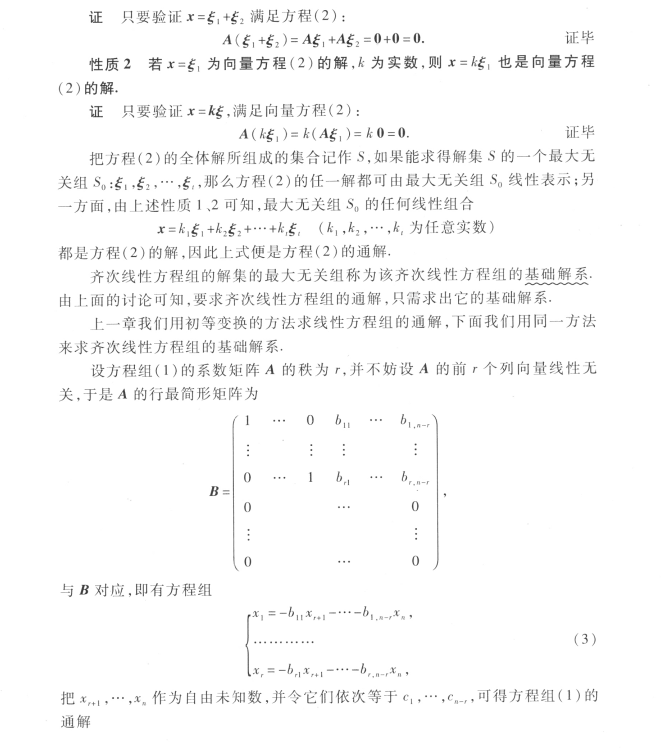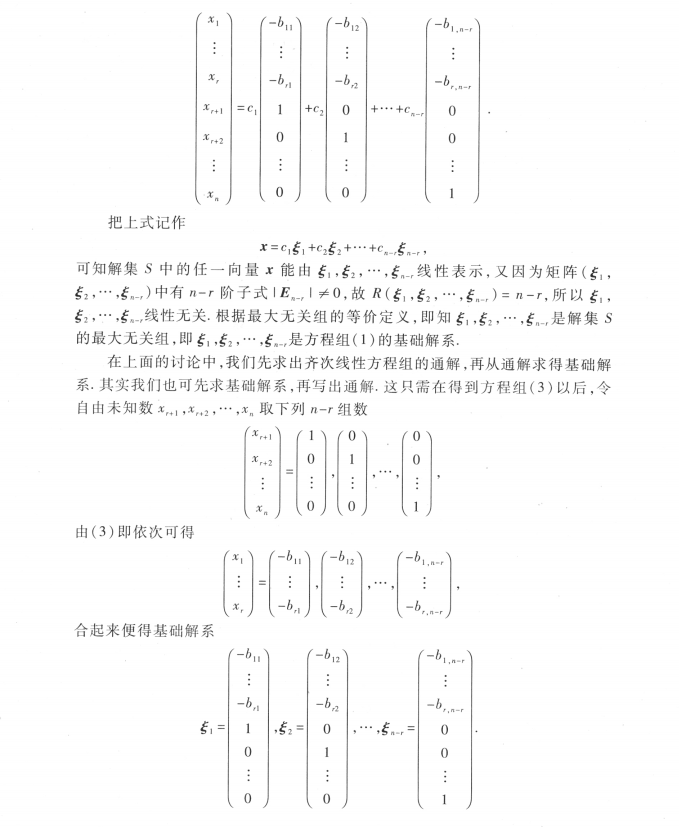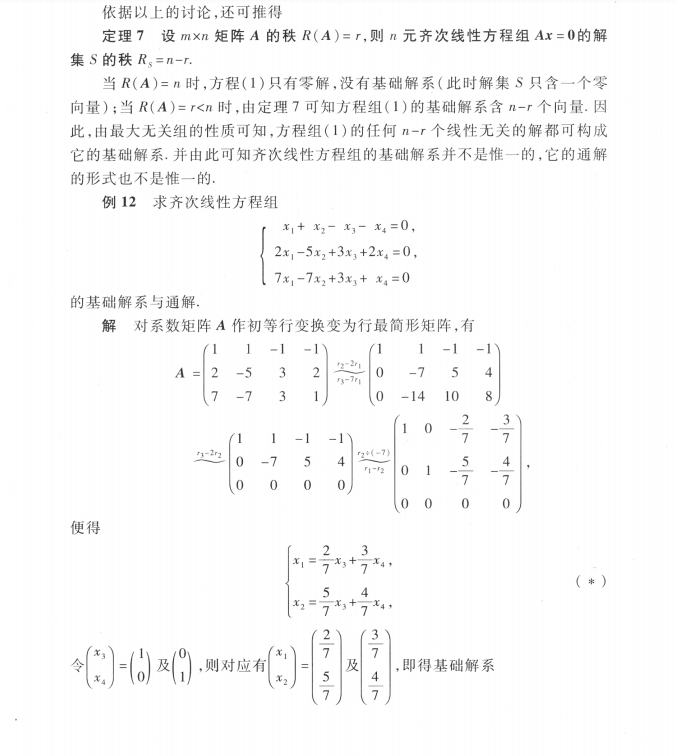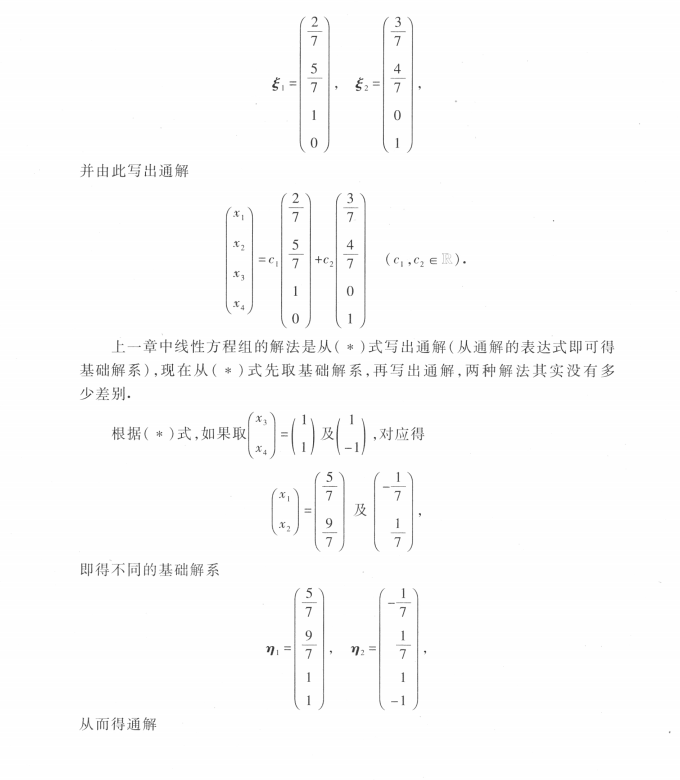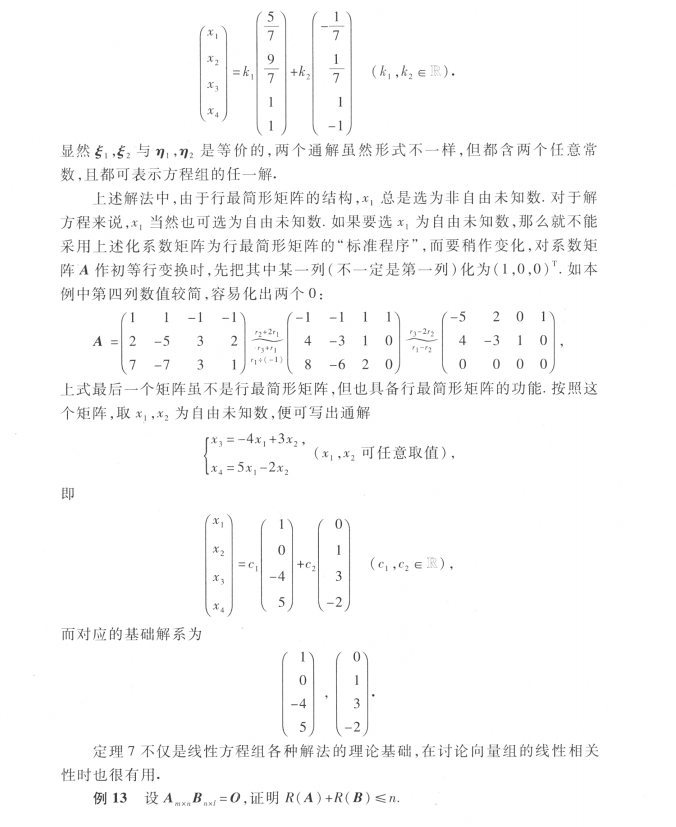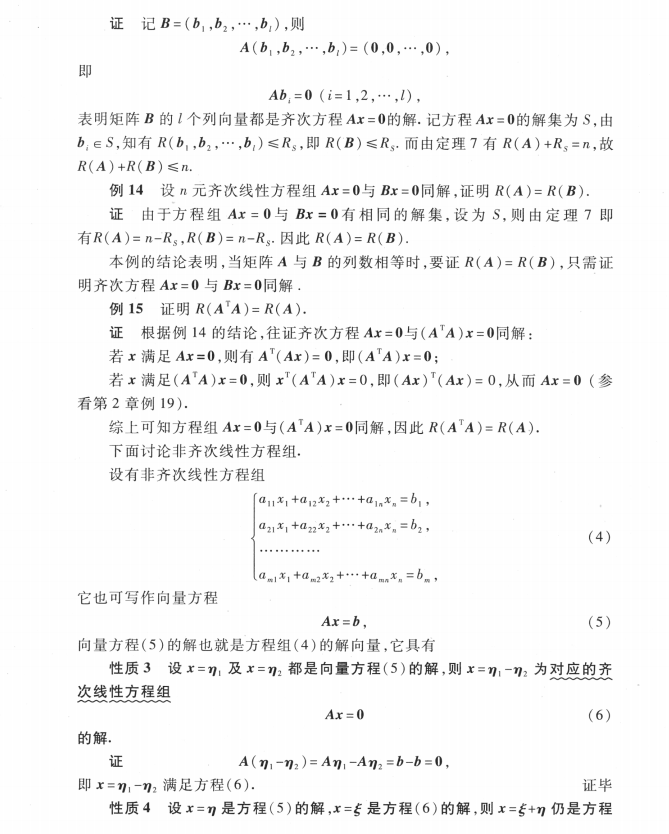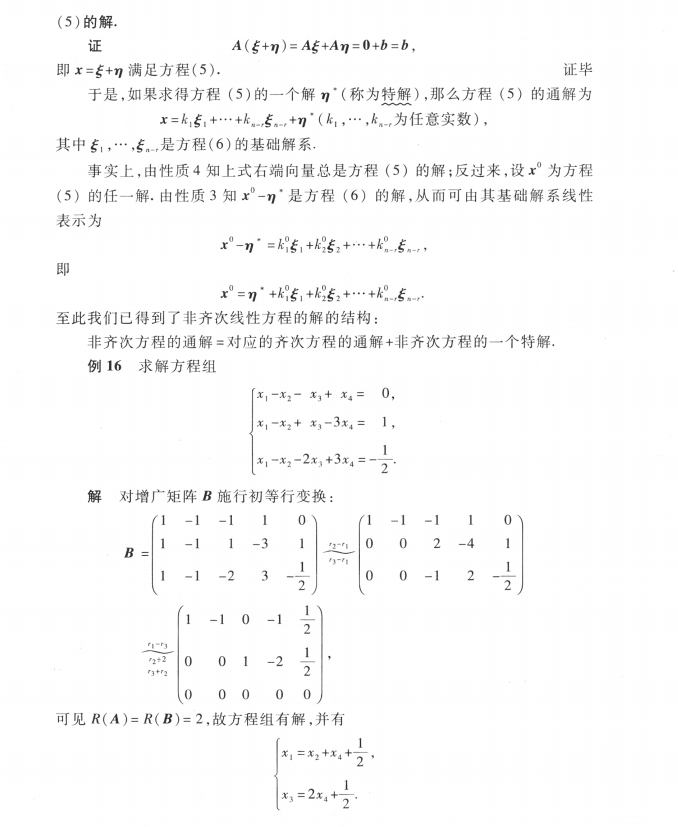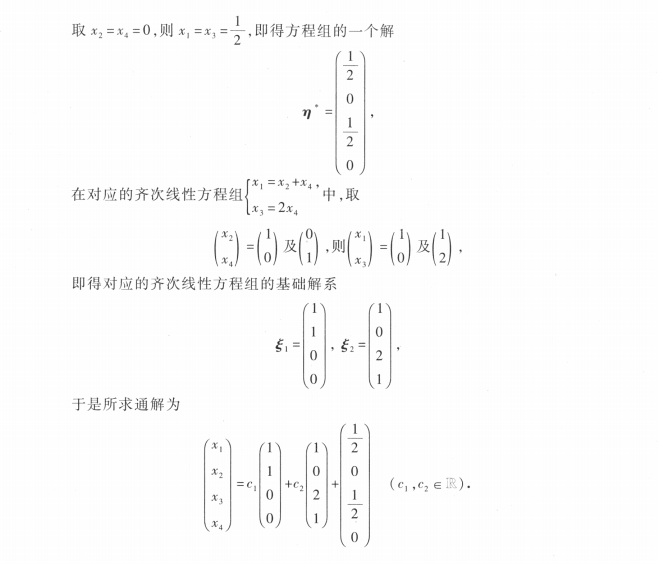展开全文• 4.4非齐次线性方程组解结构 导出组 首先 Ax = b是一个非齐次线性方程组，若Ax = 0，则叫这个齐次方程组为导出组 性质 若a1,a2是Ax = b的解，则a1 - a2 是Ax = 0的解，即非齐次方程组的解相减得到齐次方程组的解 ...c++ 容器 开发语言
• 文章目录齐次线性方程组解结构解的性质解空间基础解系基础解系存在性非齐次线性方程组解结构解的性质解的结构参考 齐次线性方程组解结构 {a11x1+a12x2+⋯+a1nxn=0a21x1+a22x2+⋯+a2nxn=0⋯⋯⋯⋯⋯⋯⋯⋯as1x1...
• 线性代数线性方程组解结构PPT学习教案.pptx
• 考虑线性方程组AX=b的(X∈Rn,b∈Rm)，如果方程组，则结构一定是某个特加上对应的其次方程的(G/ker(f)≅Im(f))考虑线性方程组AX=b的(X\in R^n,b\in R^m)，如果方程组，\\ 则结构一定是某个特...
• c2​,...,cn−r​, 由B(或A)的行最简形，即可写出含n-r个参数的通解 齐次方程组解结构定理 齐次方程组 Am×nX=0A_{m×n}X = 0Am×n​X=0的基础解系所含向量个数为 n−r (r=R(A))n-r \ \ \ (r=R(A))n−r (r=R(A)) ...AI 数学
• 线性代数—线性方程组解结构PPT学习教案.pptx
• 线性代数PPT课件4.4非齐次线性方程组解结构.ppt
• 线性代数ch齐次线性方程组解结构PPT学习教案.pptx
• 本文将总结关于线性方程组解的知识点。 线性方程组 定义1 线性方程组：我们将形如下式的方程组称为线性方程组。 a11x1+a12x2+⋯+a1nxn=b1a21x1+a22x2+⋯+a2nxn=b2…am1x1+am2x2+⋯+amnxn=bm(21)(21)a11x1+a12...
• 线性方程组{a11x1+a12x2+⋯+a1nxn=b1a21x1+a22x2+⋯+a2nxn=b2⋯⋯⋯⋯⋯⋯⋯⋯⋯an1x1+an2x2+⋯+annxn=bn\begin{cases} a_{11}x_{1} + a_{12}x_{2} + \cdots +a_{1n}x_{n} = b_{1} \\ a_{21}x_{1} + a_{22}x_{2} + \...matrix
• 考虑到弹性梁受热效应的影响,本文研究的是具有转动惯量和结构阻尼及非线性外阻尼的非自治热弹耦合梁方程组的初边值问题。根据对阻尼项和与时间有关的连续外力项的假设,采用Galerkin方法,结合先验估计,证明了方程组...
• 对变系数线性齐次微分方程组的特殊类型的求解问题进行了探讨，给出了系数矩阵为A（x）（各元素为工的多项式）的一阶线性齐次微分方程组解结构定理，以及系数矩阵为Af（x）（A为n阶常数矩阵，f（x）为可积函数）的...
• ## 微分方程解的结构

千次阅读 2020-09-08 12:10:41
今天复习一下微分方程解结构 文章目录一阶微分方程可分离的变量方程齐次方程可化为齐次的方程（可适用于更一般的方程） tips: 1.微分方程指的是：含有未知函数及其导数的方程。 2.阶数取决于方程中出现的最高次...
• 研究了一类具有结构阻尼的耦合梁方程组的初边值问题,运用Galerkin方法证明了方程组弱解和强存在的唯一性,以及对初值的连续依赖性。
• 一维零压流体运动方程组的局部结构，赵引川，张茜，本文主要研究的是一维零压流体运动方程组，通过引进势函数并讨论它的最小值点，当初值(x,t) ∈R×(0,∞) 时，得出的局部结构的以下�
• 利用正交化列处理法和线性变换，给出了一个确定任意齐次线性代数方程组解空间结构的数值计算方法，分析了该方法的收敛性、计算复杂度、数值稳定性和内在并行性，进而探讨了该方法的应用前景．
• Javascript写的数据结构实验 多项式运算及线性方程组求解，高分代码
• 本章主要介绍向量空间的知识，与前两章一样本章也可以通过研究线性方程组把所有知识点串联起来，比如研究齐次线性方程组可以得到线性相关、线性无关、零空间、空间的基（基础系）、空间的维数、秩...
• 文章目录一、二元一次方程组解析 一、二元一次方程组解析
• 以图形，数值和分析方式求解一系列形式为的指数方程。 分析解决方案是根据Lambert-W函数得出的。 对于任何y，都可以用n或以y为底的通用数值。... 根据基本常数推导了提供作为精细结构常数的方程式。
• 研究了一类具有非局部源的反应扩散方程组的初边值问题，首先利用上下方法，构造出不同结构的上解，结合比较原理，得到的整体存在的充分条件，其次利用特征函数和常微分方程的，构造问题在有限时刻爆破的下，...
• 利用推广的(w/g)展开法，并借助于计算机代数系统maple，获得了(2+1)维破裂孤子方程组新的显式，包括单循环孤立子、三角周期、有理函数等。...